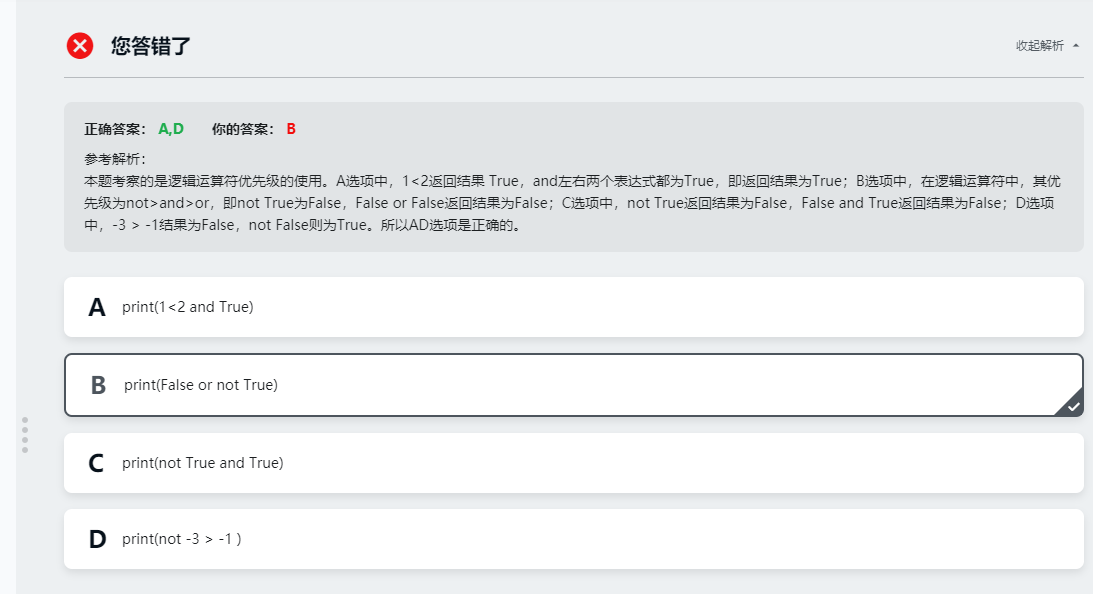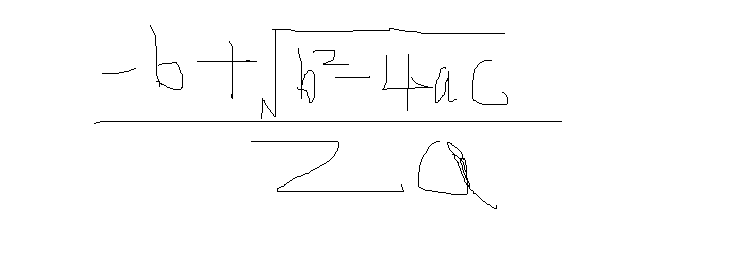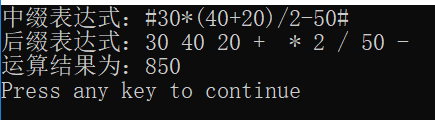• 请写出下列表达式，并编写程序验证。


(1) 3.5*3+2*7-'a'
(2) 26/3+34%3+2.5
(3) 45/2+(int)3.14159/2
(4) a=b=(c=a+=6)
设 a
的初值为 3
(5) a=3*5,a=b=3*2
(6) (int)(a+6.5)%2+(a=b=5) 设
a 的初值为
3
(7) x+a%3*(int)(x+y)%2/4 设
x=2.5,a=7,y=4.7(8)(float)(a+b)/2+(int)x%(int)y
设 a=2,b=3,x=3.5,y=2.5

#include <iostream>

using
namespace std;

int main()

{

cout <<  3.5*3+2*7-'a'
<< endl;

cout <<  26/3+34%3+2.5
<< endl;

cout <<  45/2+(int)3.14159/2
<< endl;

int a =
3,b,c;

cout << (a=b=(c=(a+=6))) << endl;

cout<< (a=3*5) <<endl;

cout<< (a=b=3*2) <<endl;

a=3;

cout <<   (int)(a+6.5)%2+(a=b=5)
<< endl;

int x=2.5,y=4.7;a=7;

cout <<  x+a%3*(int)(x+y)%2/4
<< endl;

a=2,b=3,x=3.5,y=2.5;

cout <<  (float)(a+b)/2+(int)x%(int)y
<< endl;

return
0;

}

打印：

-72.5
11.5
23
9
15
6
6
2
3.5


展开全文• and左右两个表达式True，即返回结果True； B选项中， 在逻辑运算符中，其优先级not>and>or，即not TrueFalse， False or False返回结果False； C选项中， not True返回结果False，F...
正确答案： A,D 你的答案： B
参考解析：

本题考察的是逻辑运算符优先级的使用。
A选项中，1<2返回结果 True，
and左右两个表达式都为True，即返回结果为True；
B选项中，
在逻辑运算符中，其优先级为not>and>or，即not True为False，
False or False返回结果为False；
C选项中，
not True返回结果为False，False and True返回结果为False；展开全文练习
• 2.6 package booksTest; import seventh.ScannerFileTest; ...import java.util.Scanner;... public static void main(String... 编写程序 ，要求用户从键盘输入a 、b 、和c的，计算下列表达式 */ Scanner sc =
2.6package booksTest;

import seventh.ScannerFileTest;

import java.util.Scanner;

public class p34_2_6 {
public static void main(String[] args) {
/*
题目要求
编写程序 ，要求用户从键盘输入a 、b 、和c的值，计算下列表达式的值
*/
Scanner sc = new Scanner(System.in);
for (int i = 0; i <10 ; i++) {
System.out.println("请输入a的值");
double a = sc.nextDouble();
System.out.println("请输入b的值");
double b = sc.nextDouble();
System.out.println("请输入c的值");
double c = sc.nextDouble();
double e = Math.sqrt(b * b - 4 * a * c);
double f = -b + e;
if (e > 0) {
System.out.println("表达式的值为" + f / 2 * a);
return;
} else {
System.out.println("你输入的值b^2-4ac小于0");
}
}
}
}


展开全文• 要求：设计一个算法，将一般算术表达式转化逆波兰表达式，并求逆波兰表达式。 实现思路 获取一个中缀表达式表达式转换后缀表达式 计算后缀表达式的结果 中缀表达式转换后缀表达式的几个关键部分 ...
要求：设计一个算法，将一般算术表达式转化为逆波兰表达式，并求逆波兰表达式的值。

实现思路

获取一个中缀表达式
将表达式转换为后缀表达式
计算后缀表达式的结果
中缀表达式转换为后缀表达式的几个关键部分

假如不是运算符，则输出，否则进行下面步骤

假如遇到空栈或者‘(’时，直接入栈，并继续，因为第一个遇到的肯定是'#'，所以直接入栈。
假如遇到'#'，则说明表达式结束了，但得在前一点的后面进行判断。
假如遇到')'，则进行出栈，知道遇到'('，并弹出，但不记录，因为我们不要括号的
优先级比较：
假如优先级大于栈顶，则入栈
小于或等于栈顶时，一直出栈，直到当前运算符优先级大于栈顶，则把当前运算符进栈。
计算后缀表达式

前一步已经将表达式存放在新的数组里面了，并且为了方便识别，在适当的位置都加了空格，方便识别。

由于需要进行计算，且要把char的数转换成一个整数，所以这里简单的利用一个整型数组，并且模拟栈的实现方式，基本思想如下：

对获取到后缀表达式进行扫描
如果为数字，则继续扫描直到遇到空格，并把数入栈。
假如为运算符，则进相应的运算，从栈中取出离栈顶最近的两个数，进行计算，并且把结果写在离栈顶较远的那个位置，并且把当前栈顶的数据清零。
最后获取到的结果会存放在数组的0位置。
结语

由于这个实验是我在学数据结构时的一个实验，刚学习编程，语言也比较混乱，如有错误的地方，欢迎指出。以下为代码部分：

#include <stdio.h>
#include <string.h>
#include <stdlib.h>
/*
(1)设计一个算法，将一般算术表达式转化为逆波兰表达式，并求逆波兰表达式的值。
例如 #30*(40+20)/2-50#  #为两个界限符
*/
const int StackSize = 30;
char result;//接收转换结果，并添加空格
char num;//用来result数组的计数

//定义一个栈结构体
typedef struct Stack
{
char data[StackSize];
int top;
}StackType;

//出栈处理，输入：栈结构体
char Pop(StackType *p)
{
char x;
if(p->top == -1)
{
printf("under flow\n");
exit(0);
}
x = p->data[p->top--];
return x;
}
//入栈处理
void Push(StackType *p, char dat)
{
if(p->top == StackSize -1)
{
printf("over flow\n");
exit(0);
}
p->data[++p->top] = dat;
}

//为空时返回1，不为空则返回0
bool Emtry(StackType *p)
{
if(p->top == -1)
return 1;
return 0;
}
//获取栈顶的数据
char GetTop(StackType *p)
{
if(p->top != -1)
return p->data[p->top];
return 0;
}

//判断是运算符还是运算对象
bool isNumber(char op)
{
switch(op)
{
case ' ':
case '(':
case ')':
case '+':
case '-':
case '*':
case '/':
case '#':		return 0;
default :       return 1;
}
}
//获取运算符的优先级，1为最高，且统计优先级
int priority(char ch)
{
int value= 10;
switch(ch)
{
case '(':
case ')':	value = 4;	break;//当遇到左括号时，所有的符号都会进行入栈处理，直到遇到右括号，或者内部运算输出
case '*':	value = 2;	break;
case '/':	value = 2;	break;
case '+':	value = 3;  break;
case '-':	value = 3;	break;
case '#':	value = 5;	break;
default:  break;
}
return value;
}

/*
函数名：Nifix2Postfix
输  入：数组
返  回：无
功  能：将中缀表达式转换成后缀表达式并输出
*/
void Nifix2Postfix(char arr[])
{
StackType* pStack = new StackType;
pStack->top = -1;
int i = 0;
char ch;
for(i=0;arr[i] != '\0';i++)
{
if(isNumber(arr[i]))
{
result[num++] = arr[i];
}
//不是对象时，即为运算符
else
{
//首先判断是否为空，如果为空，则入栈
if(Emtry(pStack) || arr[i] == '(')
{
Push(pStack, arr[i]);
continue;
}
if(arr[i] == '#')//结束符号
break;
if(arr[i] == ')')//出栈直到遇到(
{
result[num++] = ' ';
while((ch = Pop(pStack)) != '(' )//开始出栈，出到'('
{
result[num++] = ch;
result[num++] = ' ';
}
continue;
}
result[num++] = ' ';
//优先级比较
ch = priority(GetTop(pStack)) - priority(arr[i]);//获取优先级比较后的结果，但ch>0时，运算符优先级大于栈顶，=0相等，<0小于
//优先级大于栈顶
if(ch > 0)
{
//优先级大的入栈
Push(pStack, arr[i]);
}
//优先级小于或等于栈顶
else if(ch <= 0)
{
//应该是出栈一个，然后判断优先级，假如不为空栈，继续判断优先级，然后将arr[i]入栈
while( priority(arr[i]) >= priority(GetTop(pStack)))//此运算符优先级小于等于栈顶时，一直输出
{
//					printf("%c",ch = Pop(pStack));
result[num++] = Pop(pStack);
//					printf(" ");
result[num++] = ' ';
}
Push(pStack, arr[i]);
}

}

}
while(GetTop(pStack) != '#')
{
result[num++] = ' ';
result[num++] = Pop(pStack);
}
for(i=0;i<num;i++)
printf("%c",result[i]);
}

/*
函数功能：计算后缀表达式的值
输入：一个后缀表达式
输出：结果
*/
int Calculate(char arr[])
{
int i, cal,top=-1;
memset(cal,0,sizeof(cal));
for(i=0;i<num;i++)
{
if(isNumber(arr[i]))//判断是否是操作符，返回1不是，进来的只能是数字
{
//模拟入栈
top++;
while(arr[i] != ' ')//获取一个整数，并入栈
{
cal[top] = cal[top]*10 + arr[i++]-48;
}
}
else
{
switch(arr[i])
{
//模拟出栈
case '+':	cal[top-1] = cal[top-1] + cal[top]; cal[top--] = 0; break;
case '-':	cal[top-1] = cal[top-1] - cal[top]; cal[top--] = 0; break;
case '*':	cal[top-1] = cal[top-1] * cal[top]; cal[top--] = 0; break;
case '/':	cal[top-1] = cal[top-1] / cal[top]; cal[top--] = 0; break;
default: break;
}
}
}
return cal;
}

int main(void)
{
char  express[] = "#30*(40+20)/2-50#";

//	gets(express);

printf("中缀表达式：");
printf("%s\n", express);

printf("后缀表达式：");
Nifix2Postfix(express);
printf("\n");

printf("运算结果为：%d\n", Calculate(result));

return 0;
}



运行结果：展开全文• /*编制程序，输入m、n（m≥n≥0）后，计算下列表达式并输出。 要求将计算阶乘的运算编写作函数fact(n)，函数返回值的类型float*/#include<stdio.h> double fact(int j); int main(void) { int m,n;...
• 中缀表达式转后缀表达式：中缀表达式转后缀表达式遵循以下原则： 1.遇到操作数，直接输出； 2.栈空时，遇到运算符，入栈； 3.遇到左括号，将其入栈； 4.遇到右括号，执行出栈操作，并将出栈的元素输出，...
• 条件表达式值为真，程序和打印“x大于5″。逻辑错误可以很容易或很难找到，根据问题的性质。 另一个常见的语义错误是违反假设。一违反假设发生时，程序员的假设的东西都将是真实的或虚假的，而不对。例如： ...
• result=%.0f\n " ,y); return 0 ; } double fact( int n) { int i; double product; product = 1 ; for (i= 1 ;i;i++ ){ product =product* i; } return product; } 转载于:...
• ## 中缀表达式转换为后缀表达式

千次阅读 多人点赞 2019-07-08 17:31:58
一、什么要用后缀表达式 将中缀表达式转换成等价的后缀表达式后，求时，不需要再考虑运算符的优先级，只需从左到右扫描一遍后缀表达式即可 二、后缀表达式 后缀表达式也叫做逆波兰表达式，其求过程...算法
• 1）递归执行下列步骤即可求：先分别求出左子树和右子树表示的子表达式，最后根据根结点的运算符的要求，计算出表达式的最后结果。 2）二叉树的中序遍历序列与原算术表达式基本相同，但是需要将中序序列加上...数据结构
• ## 计算表达式的值

千次阅读 2017-08-20 21:15:51
描述 有些语言中表达式的运算符使用字符串表示，例如用mul代表*，用div代表/，用add代表+...输出n行，每行是对应表达式。注意，此处要求的所有运算均整数运算。 样例输入 5 345 mul 122 3945 div 12 321 ad
• 　中缀表达式的求问题是一个比较常见的问题之一，我们通常在编写程序时，直接写出表达式让编译器去处理，很少去关心编译器是怎么对表达式进行求的，今天我们来一起了解一下其中具体的原理和过程。 　表达式
• 假设算术表达式只包含”+”、“-”、“*”、“/”、正整数和括号的合法数学表达式，求算术表达式值。 过程是：先将算术表达式转换成后缀表达式(逆波兰式)，然后对该后缀表达式求值。 　1)将算术表达式exp转换成...
• 解决表达式首先需要根据字符串表达式求出后缀表达式，然后使用后缀表达式和操作数栈实现计算， 计算的大致思想是从后缀表达式中取元素，如果元素是数值则加入到操作数栈中， 如果是运算符则从操作数栈中取两...
• 现在已经有很多开源可用的 java 表达式引擎,什么还需要 Avaitor 呢? Aviator的设计目标是轻量级和高性能,相比于Groovy、JRuby的笨重, Aviator非常小, 加上依赖包也才 537K,不算依赖包的话只有 70K; 当然, ...Aviator scala
• 假设算术表达式的语义二叉树已经给出，请编码实现算术表达式的中缀形式（保持计算语义）的输出，并计算出该表达式。 要求： 1）使用二叉树的完全前序序列建立表达式的语义二叉树，空子树用符号@表示； 2）算术...数据结构 数据结构与算法实验
• 源代码 #include<stdio.h> #include<stdlib.h>...#define ERROR 0 #define STACKINCREMENT 5 #define STACK_INIT_SIZE 10 typedef char SElemType; typedef int Status; typedef struct{ SEle...数据结构作业 实习报告
• ## 算术表达式求值（C语言栈）

千次阅读 多人点赞 2020-11-16 15:24:08
题目：算术表达式 题目描述：表达式计算是实现程序设计语言的基本问题之一，也是栈的应用的一个典型例子。设计一个程序，演示用运算符优先法对算数表达式的过程。 基本要求：以字符序列的形式从终端输入...算法 数据结构
• 数据结构 表达式 在计算机中，表达式可以有三中不同的标识方法 设 Exp ＝ S1 + OP + S2 则， 称 op + S1 + S2 为表达式的 “前缀表达式” 称 S1 + OP + S2 为表达式的 “中缀表达式” 称 S1 +...
• 我们知道，在C 语言中经常使用 诸如 if（表达式）{} ，while (表达式) {} 形式的判断语句，当表达式为真时执行 {} 中的函数体，但是当表达式为赋值表达式时怎么判断该表达式为真还是假？或者当表达式为逗号表达式...
• 今天在论坛里面逛发现一个问题比较典型，我们在使用js的时候可以直接使用Eval得到某一个表达式，在C#里面可以吗？答案是肯定的，在.NET提供的丰富的类库里面这是可以实现的，但是没有js那么简单下面我将使用一个...
• 中缀表达式就是我们日常生活中使用的习惯性的表达式，例如：1 + 2 * 3，这里我们实现从键盘接受一段中缀表达式，并对其进行求，输出结果。 具体步骤如下： 首先从键盘接受一段中缀表达式。使用Java 栈的应用
• 一般来说，为了算法的简化和代码可读性方面的考虑，都是把我们所习惯写的中缀表达式转化后缀表达式后，再进行相关的计算，各种计算器程序的原理也与之类似。 本次博客主要讲述两个方面的内容：中缀表达式向后缀...
• Lambda 表达式使用Lambda 运算符 “=>”来定义，语法如下： (参数列表) => {方法体} Lambda 运算符的左边是输入参数，定义Lambda表达式的接收参数列表，右边包含表达式或语句块，表示将表达式或语句块返回的...c# Lambda
• 中缀表达式转换后缀表达式 1.创建栈 2.从左向右顺序获取中缀表达式 a.数字直接输出 b.运算符 情况一：遇到左括号直接入栈，遇到右括号将栈中左括号之后入栈的运算符全部弹栈输出，同时左括号出栈但是不输出。...
• 假定待求的后缀表达式为：6 5 2 3 + 8 * + 3 + *，则其求过程如下： 1）遍历表达式，遇到的数字首先放入栈中，此时栈如下所示： 2）接着读到“+”，则弹出3和2，执行3+2，计算结果等于5，并将5压入到...
•c语言
• 例题：下列给定程序中,函数fun的功能是:通过某种方式实现两个变量的交换,规定不允许增加语句或表达式。 例如变量x中的1,y中的2,程序运行后x中的值为2,y中的值为1。 注意:不要改动main函数,不能增行或...
• 给出一个后缀表达式，如 358*2/+6- （中缀表达式为3+5*8/2-6，结果17），写程序求出它的。 A: 首先想到用栈来解决问题，当读到数字时取出放入栈中，读到运算符时则从栈中取出两个数进行运算并将结果存到栈...java...